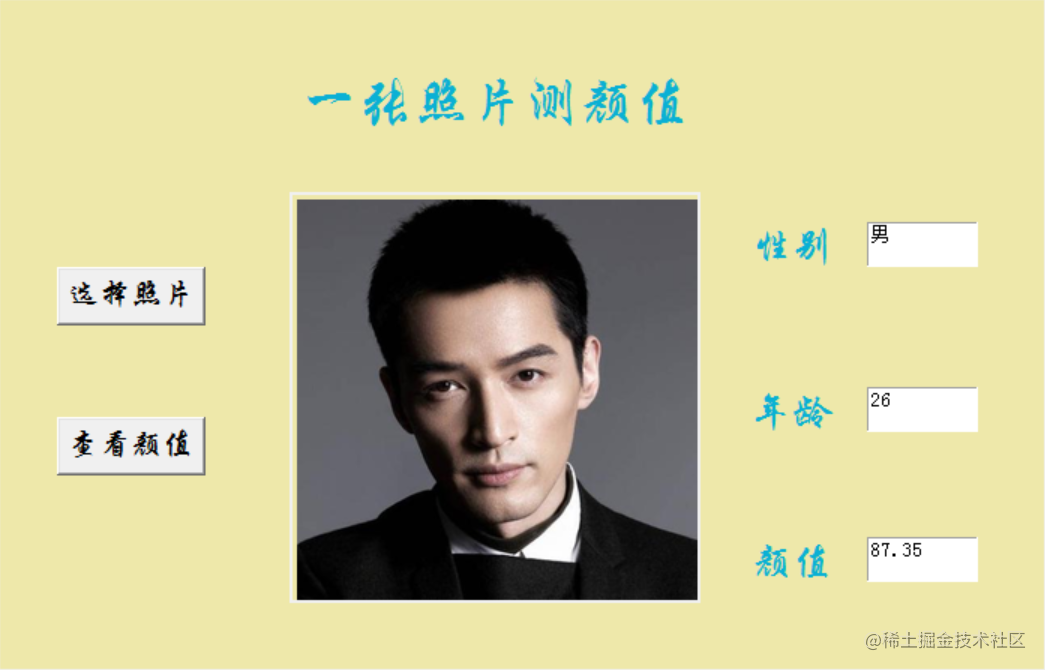# 用 Python 写一个颜值测试小工具

## 实现

``````APP_ID = '自己的APP_ID'
API_KEY = '自己的API_KEY'
SECRET_KEY = '自己的SECRET_KEY'
face = AipFace(APP_ID, API_KEY, SECRET_KEY)
image_type = 'BASE64'
options = {'face_field': 'age,gender,beauty'}

def get_file_base64(file_path):
with open(file_path, 'rb') as fr:
return content.decode('utf8')

def get_score(file_path):
# 脸部识别分数
result = face.detect(get_file_base64(file_path), image_type, options)
# print(result)
age = result['result']['face_list']['age']
beauty = result['result']['face_list']['beauty']
gender = result['result']['face_list']['gender']['type']
return age, beauty, gender

``````root = tk.Tk()
# 设置窗口大小
root.geometry('700x450')
# 为窗口添加标题
root.title('颜值测试工具')
# 设置背景色
canvas = tk.Canvas(root,
width=700,
height=450,
bg='#EEE8AA')
canvas.pack()

``````# 照片选择按钮
tk.Button(self.root, text='选择照片', font=('华文行楷', 16), command=self.show_img).place(x=40, y=180)
# 颜值测试按钮
tk.Button(self.root, text='查看颜值', font=('华文行楷', 16), command=self.set_score).place(x=40, y=280)

``````tk.Label(self.root, text='性别', bg='#EEE8AA', fg='#0AB0D5', font=('华文行楷', 20)).place(x=500, y=150)
self.text1 = tk.Text(self.root, width=10, height=2)
tk.Label(self.root, text='年龄', bg='#EEE8AA', fg='#0AB0D5', font=('华文行楷', 20)).place(x=500, y=260)
self.text2 = tk.Text(self.root, width=10, height=2)
tk.Label(self.root, text='颜值', bg='#EEE8AA', fg='#0AB0D5', font=('华文行楷', 20)).place(x=500, y=360)
self.text3 = tk.Text(self.root, width=10, height=2)
# 填装文字
self.text1.place(x=580, y=150)
self.text2.place(x=580, y=260)
self.text3.place(x=580, y=360)